# The LIFEREG Procedure

## Overview: LIFEREG Procedure

The LIFEREG procedure fits parametric models to failure time data that can be uncensored, right censored, left censored, or interval censored. The models for the response variable consist of a linear effect composed of the covariates and a random disturbance term. The distribution of the random disturbance can be taken from a class of distributions that includes the extreme value, normal, logistic, and, by using a log transformation, the exponential, Weibull, lognormal, log-logistic, and three-parameter gamma distributions.

The model assumed for the responseis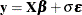whereis a vector of response values, often the log of the failure times,is a matrix of covariates or independent variables (usually including an intercept term),is a vector of unknown regression parameters,is an unknown scale parameter, andis a vector of errors assumed to come from a known distribution (such as the standard normal distribution). If an offset variable O is specified, the form of the model is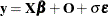, where
is a vector of values of the offset variable O. The distribution might also depend on additional shape parameters. These models are equivalent to accelerated failure time models when the log of the response is the quantity being modeled. The effect of the covariates in an accelerated failure time model is to change the scale, and not the location, of a baseline distribution of failure times.

The LIFEREG procedure estimates the parameters by maximum likelihood with a Newton-Raphson algorithm. PROC LIFEREG estimates the standard errors of the parameter estimates from the inverse of the observed information matrix.

The accelerated failure time model assumes that the effect of independent variables on an event time distribution is multiplicative on the event time. Usually, the scale function is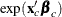, where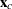is the vector of covariate values (not including the intercept term) andis a vector of unknown parameters. Thus, if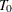is an event time sampled from the baseline distribution corresponding to values of zero for the covariates, then the accelerated failure time model specifies that, if the vector of covariates is, the event time is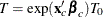. If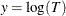and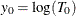, then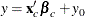This is a linear model with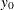as the error term.

In terms of survival or exceedance probabilities, this model is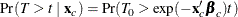The probability on the left-hand side of the equal sign is evaluated given the valuefor the covariates, and the right-hand side is computed using the baseline probability distribution but at a scaled value of the argument. The right-hand side of the equation represents the value of the baseline survival function evaluated at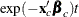.

Models usually have an intercept parameter and a scale parameter. In terms of the original untransformed event times, the effects of the intercept term and the scale term are to scale the event time and to raise the event time to a power, respectively. That is, if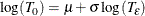then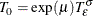Although it is possible to fit these models to the original response variable by using the NOLOG option, it is more common to model the log of the response variable. Because of this log transformation, zero values for the observed failure times are not allowed unless the NOLOG option is specified. Similarly, small values for the observed failure times lead to large negative values for the transformed response. The NOLOG option should be used only if you want to fit a distribution appropriate for the untransformed response, such as the extreme value instead of the Weibull. If you specify the normal or logistic distributions, the responses are not log transformed; that is, the NOLOG option is implicitly assumed.

Parameter estimates for the normal distribution are sensitive to large negative values, and care must be taken that the fitted model is not unduly influenced by them. Large negative values for the normal distribution can occur when fitting the lognormal distribution by log transforming the response, and some response values are near zero. Likewise, values that are extremely large after the log transformation have a strong influence in fitting the Weibull distribution (that is, the extreme value distribution for log responses). You should examine the residuals and check the effects of removing observations with large residuals or extreme values of covariates on the model parameters. The logistic distribution gives robust parameter estimates in the sense that the estimates have a bounded influence function.

The standard errors of the parameter estimates are computed from large sample normal approximations by using the observed information matrix. In small samples, these approximations might be poor. See Lawless (2003) for additional discussion and references. You can sometimes construct better confidence intervals by transforming the parameters. For example, large sample theory is often more accurate for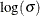than. Therefore, it might be more accurate to construct confidence intervals forand transform these into confidence intervals for. The parameter estimates and their estimated covariance matrix are available in an output SAS data set and can be used to construct additional tests or confidence intervals for the parameters. Alternatively, tests of parameters can be based on log-likelihood ratios. See Cox and Oakes (1984) for a discussion of the merits of some possible test methods including score, Wald, and likelihood ratio tests. Likelihood ratio tests are generally more reliable for small samples than tests based on the information matrix.

The log-likelihood function is computed using the log of the failure time as a response. This log likelihood differs from the log likelihood obtained using the failure time as the response by an additive term of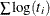, where the sum is over the uncensored failure times. This term does not depend on the unknown parameters and does not affect parameter or standard error estimates. However, many published values of log likelihoods use the failure time as the basic response variable and, hence, differ by the additive term from the value computed by the LIFEREG procedure.

The classic Tobit model also fits into this class of models but with data usually censored on the left. The data considered by Tobin (1958) in his original paper came from a survey of consumers where the response variable is the ratio of expenditures on durable goods to the total disposable income. The two explanatory variables are the age of the head of household and the ratio of liquid assets to total disposable income. Because many observations in this data set have a value of zero for the response variable, the model fit by Tobin is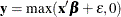which is a regression model with left censoring, where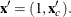Bayesian analysis of parametric survival models can be requested by using the BAYES statement in the LIFEREG procedure. In Bayesian analysis, the model parameters are treated as random variables, and inference about parameters is based on the posterior distribution of the parameters, given the data. The posterior distribution is obtained using Bayes’ theorem as the likelihood function of the data weighted with a prior distribution. The prior distribution enables you to incorporate knowledge or experience of the likely range of values of the parameters of interest into the analysis. If you have no prior knowledge of the parameter values, you can use a noninformative prior distribution, and the results of the Bayesian analysis will be very similar to a classical analysis based on maximum likelihood. A closed form of the posterior distribution is often not feasible, and a Markov chain Monte Carlo method by Gibbs sampling is used to simulate samples from the posterior distribution. See Chapter 7: Introduction to Bayesian Analysis Procedures, for an introduction to the basic concepts of Bayesian statistics. Also see the section Bayesian Analysis: Advantages and Disadvantages in Chapter 7: Introduction to Bayesian Analysis Procedures, for a discussion of the advantages and disadvantages of Bayesian analysis. See Ibrahim, Chen, and Sinha (2001) and Gilks, Richardson, and Spiegelhalter (1996) for more information about Bayesian analysis, including guidance in choosing prior distributions.

For Bayesian analysis, PROC LIFEREG generates a Gibbs chain for the posterior distribution of the model parameters. Summary statistics (mean, standard deviation, quartiles, HPD and credible intervals, correlation matrix) and convergence diagnostics (autocorrelations; Gelman-Rubin, Geweke, Raftery-Lewis, and Heidelberger and Welch tests; and the effective sample size) are computed for each parameter, as well as the correlation matrix of the posterior sample. Trace plots, posterior density plots, and autocorrelation function plots that are created using ODS Graphics are also provided for each parameter.

The LIFEREG procedure uses ODS Graphics to create graphs as part of its output. For general information about ODS Graphics, see Chapter 21: Statistical Graphics Using ODS.